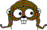Interesting Functions

Hardest n-input for (AND, OR, XOR)
(1) 1
(2) x+y = 1
(3) x+y+z = 1
(4) x+y+z+w = 1
(5) x+y+z+w+v in 0,3

Hardest n-input for (AND, OR)
(1) 1
(2) x^y
(3) x^y^z
(4) x^y^z^w
(5) x+y+z+w+v in 0,1,3,
x+y+z+w+v in 0,1,3 || x&y&z&w&!v,
x+y+z+w+v in 1,3 || x&y&z&w&!v

# Boolean Oracle

This web server computes a minimal Boolean formula for a given function, using the operator sets (AND, OR, XOR) and (AND, OR).

Query: x+y+z+w+v in 1,3 || x&y&z&w&!v (truth table 0x56696996, canonical 0x1669e996)

A minimal Boolean formula using AND, OR, and XOR requires 7 operators. One such formula is:

z ^ ¬x ^ ¬y ^ ¬v ^ (¬w | (x & y & z))

A minimal Boolean formula using AND and OR requires 28 operators. One such formula is:

((¬v | z) & (x | ((¬y | ¬w) & (y | w))) & ((w & y & ¬v) | ((v | ¬z) & (¬x | (¬y & ¬w))))) | ((v | z) & (¬x | ((¬y | ¬w) & (y | w))) & (¬v | ¬z) & (x | (y & w) | (¬y & ¬w)))

How does this work?